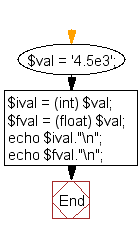﻿ PHP Math Exercise: Convert scientific notation to an int and a float - w3resource# PHP Math Exercises: Convert scientific notation to an int and a float

## PHP math: Exercise-4 with Solution

Write a PHP script to convert scientific notation to an int and a float.

Sample scientific notation : 4.5e3

Sample Solution:

PHP Code:

``````<?php
\$val = '4.5e3';
\$ival = (int) \$val;
\$fval = (float) \$val;
echo \$ival."\n";
echo \$fval."\n";
?>
```
```

Sample Output:

```4
4500
```

Flowchart :PHP Code Editor:

Have another way to solve this solution? Contribute your code (and comments) through Disqus.

What is the difficulty level of this exercise?

﻿

## PHP: Tips of the Day

SQL injection that gets around mysql_real_escape_string()

Consider the following query:

```\$iId = mysql_real_escape_string("1 OR 1=1");
\$sSql = "SELECT * FROM table WHERE id = \$iId";
```

mysql_real_escape_string() will not protect you against this. The fact that you use single quotes (' ') around your variables inside your query is what protects you against this. The following is also an option:

```\$iId = (int)"1 OR 1=1";
\$sSql = "SELECT * FROM table WHERE id = \$iId";
```

Ref : https://bit.ly/32q3bJ7